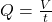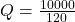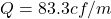Question

In a building with 10.000 cubic feet where the air changes every two hours, what the rate of air change? A. 167.7 cfm B. 83.3 cfm C. 133.3 cfm D. 50 cfm

1.bonexptip

Flow rate of air is given as 83.33 cubic feet per minute

Explanation:

As we know that total volume of the air flow is given as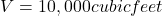also we know that total time is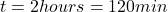now we have flow rate given as